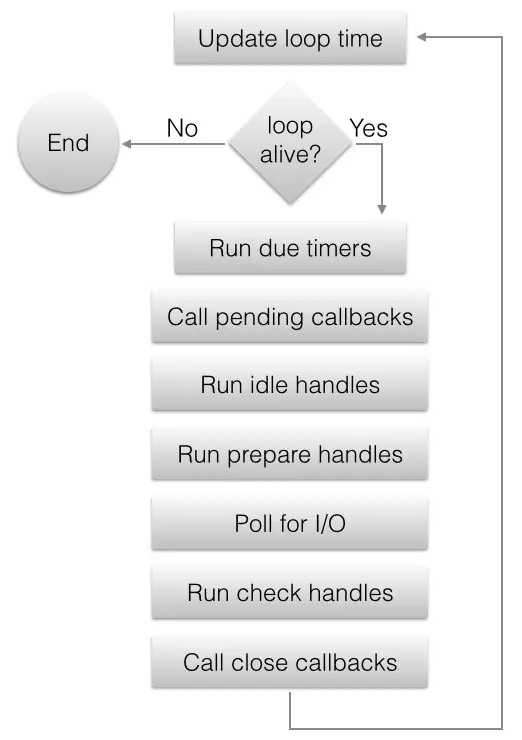# Nodejs监控事件循环异常示例详解_婳祎_前端开发者

event loop 的基础想必各位读者都比较熟悉了。这里我引用官方的图，简单介绍两句，作为前置准备：event loop是作为单线程实现异步的方式之一。简而言之，就是在一个大的 while 循环中不断遍历这些 phase，执行对应的 callbacks。这样才实现了真正的异步调用：调用时不必等着响应，等调用的资源准备好了，回调我。

1. js 既然是单线程，那么总有办法 block 住整个程序，虽然用了 libuv，也可能会 block 住主程序。对吗？
2. 如何知道我们的程序 block 住了？

```// 环境准备
const http = require('http');
const path = require('path');
const {execFile, execFileSync} = require('child_process');

const max = 9999;
const getComputedValueFromChildProcess = (max) => execFileSync('node', [path.join(__dirname, './childprocess.js'), max]);

http.createServer((req, res) => {
const k = getComputedValueFromChildProcess(max);
res.write('origin-text: ' + k);
res.end();
}).listen(8888);

// 第一版实现
const MS_MULTI = 1000 * 1000;
const blockDelta = 10 * MS_MULTI;
let start;
function meature() {
start = process.hrtime();
setImmediate(function() {
let seconds;
[seconds, start] = process.hrtime(start);
if (seconds * 1000 * MS_MULTI + start > blockDelta) {
console.log(`node.eventloop_blocked for \${seconds}secs and \${(start / MS_MULTI).toFixed(2)}ms.`);
}
meature();
});
}
meature();

// childprocess.js 文件
#!/use/env node
const args = Number(process.argv);
function computeIo(args) {
let k;
for (let i = 0; i < args; ++i) {
for (let j = 0; j < args; ++j) {
k = i + j;
}
}
return k;
}
console.log(computeIo(args));```

```# 没有请求前
# 等了很久出现一个15ms
➜ test node blocked.js
node.eventloop_blocked for 0secs and 15.71ms.

# 当我执行几次
curl https://www.rokub.com:8888
# 出现：
node.eventloop_blocked for 0secs and 175.60ms.
node.eventloop_blocked for 0secs and 149.92ms.
node.eventloop_blocked for 0secs and 147.25ms.```

```const EVERY_SEC_MIN_LOOPS = 1000000; // 定义每秒最小循环数
let times = 0; // 一次采样中的循环数
let nowShowIncreaseTimes = false; // 当前是否应该增加 times
let start = Date.now();
const CD = 10 * 1000; // 间隔
function meature(callback = () => {}) {
setTimeout(function() {
start = Date.now();
nowShowIncreaseTimes = true;
_inter();
setTimeout(() => {
endMeature();
meature(); // 开始预约下次采样
}, 2000);
}, CD);
}
function _inter() {
setImmediate(() => {
if (nowShowIncreaseTimes) {
++times;
return _inter();
}
});
}

function endMeature() {

const now = Date.now();
nowShowIncreaseTimes = false;
const totalMsSpan = now - start;
const everySecLoops = (times / (totalMsSpan / 1000)).toFixed(0);
if (everySecLoops < EVERY_SEC_MIN_LOOPS) {
console.log(`当前每秒循环数\${everySecLoops}`);
}
times = 0;
return everySecLoops
}
meature();```

# 当我不断：
curl https://www.rokub.com:8888
# 出现
➜  test node blocked.js

# 当我们搞事情时：
ab -c 10 -n 200 https://www.rokub.com:8888/

# 结果是这样的：
➜  test node blocked.js

```// non-blocked.js
const max = 9999;
const getComputedValueFromChildProcess = (max) => new Promise((res, rej) => {
execFile('node', [path.join(__dirname, './childprocess.js'), max], (err, stdout) => {
const valueFromChildProcess = Number(stdout);
res(valueFromChildProcess);
});
});

http.createServer(async (req, res) => {
const k = await getComputedValueFromChildProcess(max);
res.write('origin-text: ' + k);
res.end();
}).listen(8888);```

PS: 为了示范同步、异步的区别，本文用的是子进程这种方式。其实更好的应该是用 worker_thread 的方式、或者分片计算等。让我们用相同的 ab 进行测试，得到结果：

➜  test node non-blocked.js

64K